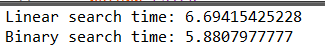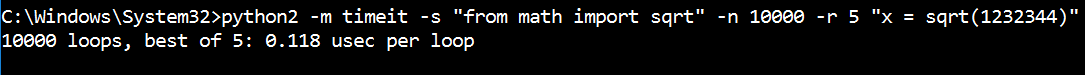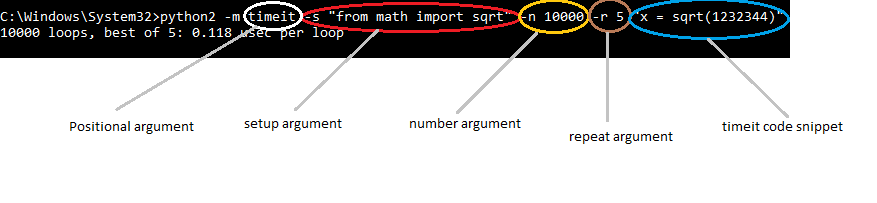Open In App

# Timeit in Python with Examples

This article will introduce you to a method of measuring the execution time of your Python code snippets. We will be using an in-built Python library timeit. This module provides a simple way to find the execution time of small bits of Python code.

## What is the use of Timeit?

Well, how about using a simple time module? Just save the time before and after the execution of code and subtract them! But this method is not precise as there might be a background process momentarily running which disrupts the code execution and you will get significant variations in the running time of small code snippets. Timeit runs your snippet of code millions of times (default value is 1000000) so that you get the statistically most relevant measurement of code execution time! Timeit is pretty simple to use and has a command-line interface as well as a callable one.

## Python Timeit() Syntax:

Syntax: timeit.timeit(stmt, setup, timer, number)

Parameter:

• stmt which is the statement you want to measure; it defaults to ‘pass’.
• setup which is the code that you run before running the stmt; it defaults to ‘pass’.
We generally use this to import the required modules for our code.
• timer which is a timeit.Timer object; it usually has a sensible default value so you don’t have to worry about it.
• the number which is the number of executions you’d like to run the stmt.

Returns the number of seconds it took to execute the code.

## Python3

 `# testing timeit()``import` `timeit` `# code snippet to be executed only once``mysetup ``=` `"5+5"` `print``(timeit.timeit(mysetup))`

Output:

`0.0237445189995924`

### Timing Multiple Lines in Python Code

There are two ways to run numerous lines of code in timeit.timeit(), a semicolon, or by saving the code wrapped in triple quotes as a string.

Example 1: Using a semicolon

## Python3

 `# testing timeit()``import` `timeit` `# code snippet to be executed``print``(``"The time taken is "``,timeit.timeit``                      ``(stmt``=``'x=15;y=15;sum=x+y'``))`

Output:

`The time taken is  0.06830515900219325`

Example 2: Using triple Quotes

The program is pretty straightforward. All we need to do is to pass the code as a string to the timeit.timeit() function. It is advisable to keep the import statements and other static pieces of code in the setup argument.

## Python3

 `# importing the required module``import` `timeit` `# code snippet to be executed only once``mysetup ``=` `"from math import sqrt"` `# code snippet whose execution time is to be measured``mycode ``=` `'''``def example():``    ``mylist = []``    ``for x in range(100):``        ``mylist.append(sqrt(x))``'''` `# timeit statement``print``(timeit.timeit(setup``=``mysetup,``                    ``stmt``=``mycode,``                    ``number``=``10000``))`

Output:

Note: Pay attention to the fact that the output is the execution time of the number times iterations of the code snippet, not the single iteration. For a single iteration exec. time, divide the output time by a number.

`0.0024192919954657555`

### Example 3: Using  timeit.repeat()

Let’s see another practical example in which we will compare two searching techniques, namely, Binary search and Linear search.  Also, here we demonstrate two more features, timeit.repeat function and call the functions already defined in our program.

## Python3

 `# importing the required modules``import` `timeit` `# binary search function``def` `binary_search(mylist, find):``    ``while` `len``(mylist) > ``0``:``        ``mid ``=` `(``len``(mylist))``/``/``2``        ``if` `mylist[mid] ``=``=` `find:``            ``return` `True``        ``else` `if` `mylist[mid] < find:``            ``mylist ``=` `mylist[:mid]``        ``else``:``            ``mylist ``=` `mylist[mid ``+` `1``:]``    ``return` `False`  `# linear search function``def` `linear_search(mylist, find):``    ``for` `x ``in` `mylist:``        ``if` `x ``=``=` `find:``            ``return` `True``    ``return` `False`  `# compute binary search time``def` `binary_time():``    ``SETUP_CODE ``=` `'''``from __main__ import binary_search``from random import randint'''` `    ``TEST_CODE ``=` `'''``mylist = [x for x in range(10000)]``find = randint(0, len(mylist))``binary_search(mylist, find)'''` `    ``# timeit.repeat statement``    ``times ``=` `timeit.repeat(setup``=``SETUP_CODE,``                          ``stmt``=``TEST_CODE,``                          ``repeat``=``3``,``                          ``number``=``10000``)` `    ``# printing minimum exec. time``    ``print``(``'Binary search time: {}'``.``format``(``min``(times)))`  `# compute linear search time``def` `linear_time():``    ``SETUP_CODE ``=` `'''``from __main__ import linear_search``from random import randint'''` `    ``TEST_CODE ``=` `'''``mylist = [x for x in range(10000)]``find = randint(0, len(mylist))``linear_search(mylist, find)``    ``'''``    ``# timeit.repeat statement``    ``times ``=` `timeit.repeat(setup``=``SETUP_CODE,``                          ``stmt``=``TEST_CODE,``                          ``repeat``=``3``,``                          ``number``=``10000``)` `    ``# printing minimum exec. time``    ``print``(``'Linear search time: {}'``.``format``(``min``(times)))`  `if` `__name__ ``=``=` `"__main__"``:``    ``linear_time()``    ``binary_time()`

Output:

The output of the above program will be the minimum value in the list times.• strate below how you can utilize the command line
• timeit.repeat() function accepts one extra argument, repeat. The output will be a list of the execution times of all code runs repeated a specified no. of times.
• In the setup argument, we passed:
```from __main__ import binary_search
from random import randint```
• This will import the definition of the function binary_search, already defined in the program, and the random library function randint.
• As expected, we notice that the execution time of binary search is significantly lower than linear search!

### Example 4: default_timer()

In this example, we find the difference in time between the start and the end.

## Python3

 `import` `timeit``import` `random`  `def` `fun():``    ``return` `random.randint(``100``, ``800``)` `start ``=` `timeit.default_timer()``print``(``"The start time is :"``, start)``fun()``print``(``"The difference of time is :"``,``              ``timeit.default_timer() ``-` `start)`

### Example 5

Finally, we demonstrate below how you can utilize the command line interface of Timeit module:Here we explain each term individually:So, this was a brief yet concise introduction to Timeit module and its practical applications. It’s a pretty handy tool for Python programmers when they need a quick glance at the execution time of their code snippets.